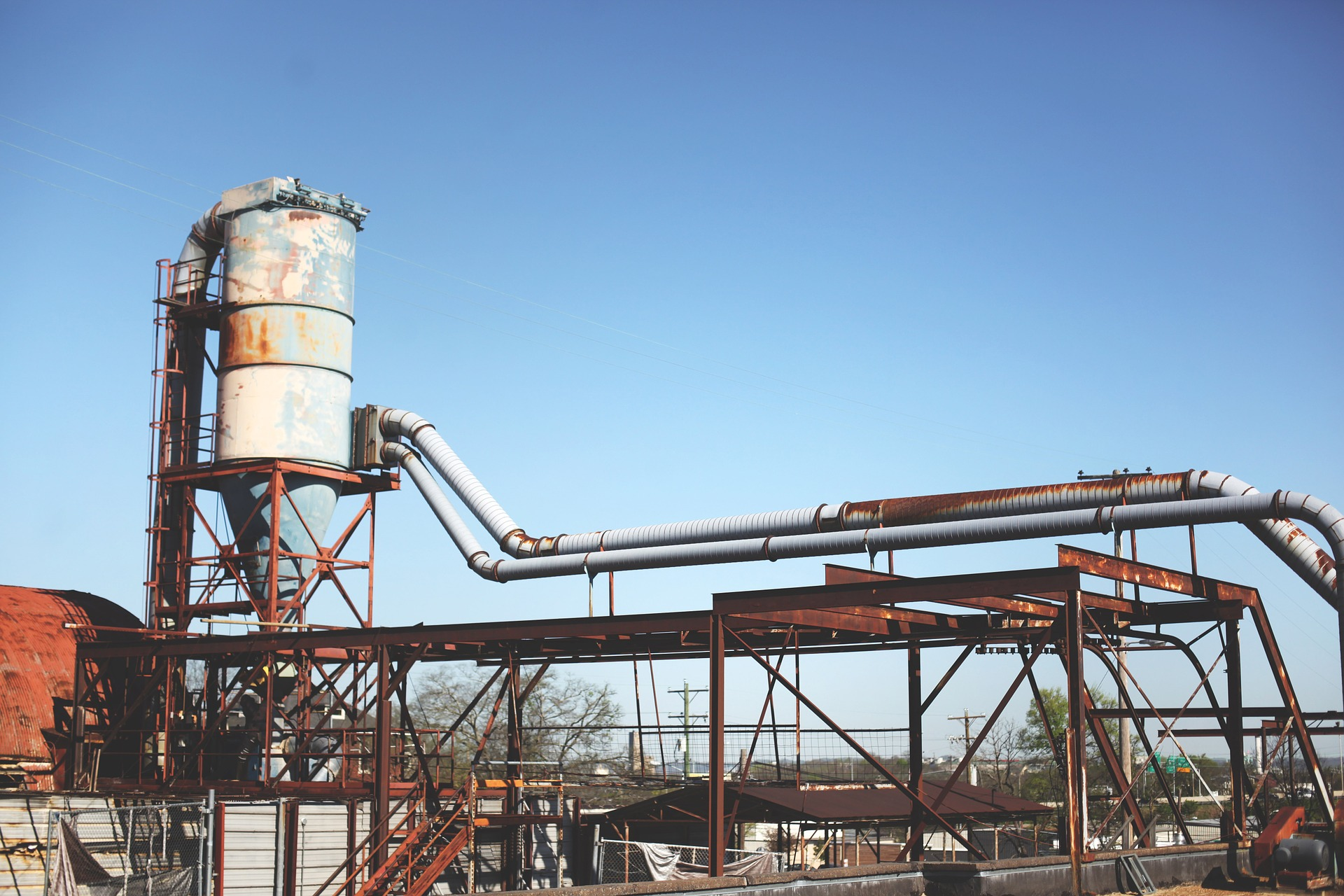# Material Balances Calculation Approach using System Boundary

In this post, I want to share how to calculate material balances using the system boundary approach. This method is especially useful when dealing with a process configuration that involves several types of equipment with a different function, such as reaction, filtration, drying, and recycling.

How to do it?

We need to divide the overall process into several process sub-division or system boundaries. The flows into and out of the system are those crossing the sub-division and must balance with material generated or consumed within the boundary.

There are no rigid rules to use this approach. Selection of the best sub-division for any process is from our judgement and by practice a lot. However, these general rules can be used as a guide to calculate material balance using the system boundary approach:

1. For complex processes, first thing to do is to take overall complete process and if possible, calculate the flows in and out. Raw materials in, products, and by-products out.
2. Select the boundaries to sub-divide the process into simple stages and make a balance over each stage separately.
3. Select the boundary round any stage to reduce the number of unknown streams to as few as possible.
4. Include any recycle streams within the system boundary.

Let’s see example below to practice.

Diagram below shows main stages in a process for polymer production. We want to calculate stream flows for a production rate of 10,000 kg/h.

The following data are known:

• Reactor yield on polymer = 100%
• Slurry polymerization = 20% monomer/water
• Conversion = 90%
• Catalyst = 1 kg/1000 kg monomer
• Short stopping agent = 0.5 kg/1000 kg unreacted monomer
• Filter, wash water = 1 kg/1 kg polymer
• Recovery column yield = 98% (percentage recovered)
• Feed to Dryer = 5% water
• Product specification = 0.5% water
• Polymer losses in filter and dryer = 1%

Solution

To solve this problem, we will use system boundary condition and determine flow in and out to the boundary. Let’s start from the product first, which is boundary of filter and dryer.

Basis: 1 hour.

Boundary #1: Filter and Dryer

In this boundary, we know this information:

• Product (polymer) = 10,000 kg
• Water content in product = 0.5% water
• Polymer losses in filter and dryer = 1%

Therefore, input to the filter and dryer = 10,000/ (100%-1%) = 10,101 kg

Take the next boundary is reactor system. Therefore, the feeds to the reactor can be calculated.

Boundary #2: Reactor

We know this information:

• Conversion = 90%
• Catalyst = 1 kg/1000 kg monomer
• Slurry polymerization = 20% monomer / water
• Short-stop agent = 0.5 kg/1000 kg unreacted monomer

Therefore, we can calculate the following:

• Input of monomer to the Reactor = 10,101/90% = 11,223 kg
• Unreacted monomer = Product outlet Reactor – monomer inlet Reactor = 11,223 – 10,101 = 1,122 kg
• Required catalyst = 1 kg x 11,223 kg / 1000 kg = 11 kg
• Water consumption = 11,223/20% – 11,223 = 44,893 kg
• Short-stop agent = 0.5 x 1,122 / 1000 = 56 kg
• Water content in feed to Dryer = 5% x 10,101 = 505 kg

Boundary #3: Reactor – Filter – Dryer

• We can estimate water to Recovery column = Water inlet – water in product = 44,893 – 505 = 44,388 kg
• Unreacted monomer is also flowing to Recovery column = 1,122 kg

Boundary #4: Recovery Column

We know this information:

• Recovery column yield = 98% (percentage recovered)

Therefore:

• Monomers recycle to reactor = unreacted monomer x recovery = 1,122 x 98% = 1,100 kg
• Assume all water is flowing to effluent
• Water to effluent = water inlet = 44,388 kg
• Monomer to effluent = unreacted monomer – monomer recycle to reactor = 1,122 – 1,100 = 22 kg

Boundary #5: Recycle Stream

Let’s not forget to include recycle stream into boundary.

From calculation above, we get:

• Reactor monomer feed = 11,223 kg (see Boundary #2)
• Monomers recycle = 1,100 kg (see Boundary #4)
• Therefore, fresh monomer feed = 11,223 – 1,100 = 10,123 kg

Summary

We, as process engineer, like to make material balance in form of table that expressed composition entering and leaving a system. Therefore, I made one based on above cases.